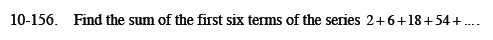Home > CCA2 > Chapter 10 > Lesson 10.3.1 > Problem10-156

10-156.

Find the sum of the first six terms of the series 2 + 6 + 18 + 54 + ... Homework Help ✎Find the common ratio.

$\frac{6}{2}=3$

Using t(n) = a · r n − 1, find t(6).

Now find the sum of the geometric series using:

$S(k)=\frac{r\cdot t(k)-t(1)}{r-1}$